# D. Dereudre: Fully-connected bond percolation on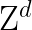Abstract: We consider the bond percolation model on the lattice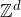() with the constraint to be fully connected. Each edge is open with probability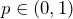, closed with probabilityand then the process is conditioned to have a unique open connected component (bounded or unbounded). The model is defined onby passing to the limit for a sequence of finite volume models with general boundary conditions. Several questions and problems are investigated: existence, uniqueness, phase transition, DLR equations. Our main result involves the existence of a threshold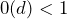such that any infinite volume process is necessary the vacuum state in subcritical regime (no open edges) and is non trivial in the supercritical regime (existence of a stationary unbounded connected cluster). Bounds for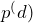are given and show that it is drastically smaller than the standard bond percolation threshold in. For instance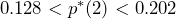(rigorous bounds) whereas the 2D bond percolation threshold is equal to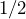.

During the talk, connections with the FK-percolation, the incipient cluster, the conjecture “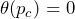” are also discussed.

## Video